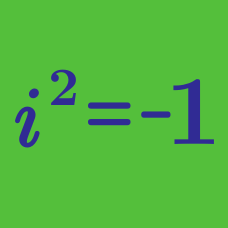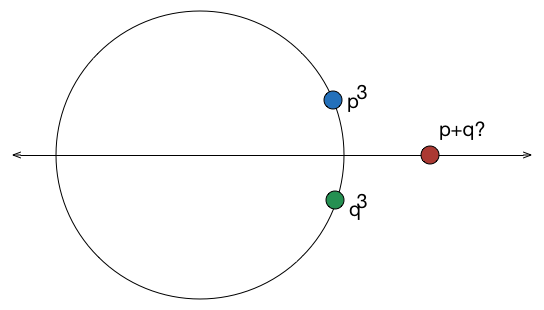Algebra

# Complex Numbers: Level 3 Challenges

If the square of $x$ and the square root of $x$ are equal to each other, but not equal to $x$, then what is the value of $x^2+x$?Let $z=x+iy$ be a complex number where $x$ and $y$ are integers. Find the area of the rectangle whose vertices are the roots of the equation $\large z \bar{z}^3+\bar{z}z^3=350.$

Details: $\bar{z}=x-iy$ and $i=\sqrt{-1}$.

How many complex numbers are the conjugate of their own cube?The complex numbers $p$ and $q$ satisfy $p^3=5+i\sqrt{2}$ and $q^3=5-i\sqrt{2}$.

Find the only possible integer value of $p+q$.

$\large e ^{ i\theta }e^{ 2i\theta} e^{ 3i\theta }...\large e^{ ni\theta } = 1$

Find the value of $\theta$ satisfying the above equation for $m\in\mathbb{N}$.

×